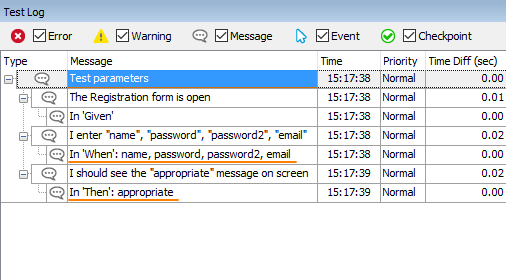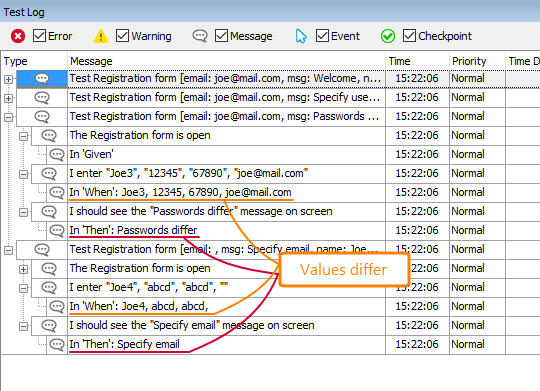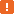# Parameterize BDD Tests### Why use parameters?

It is considered to be a good practice to run tests with different input data to check if the tested application checks incoming values in a proper way. For example, if your application has a Registration form, you may want to submit different combinations of user names, passwords and emails to check the form behavior. To test that form thoroughly, you can pass both valid and invalid values to it.

Of course, you can create different tests for each combination of values. However, a more reasonable solution is to create just one test and pass the desired values to it as parameters. Let’s see how you can do this with BDD tests in TestComplete.

### Parameterize test steps

Suppose, you need to test a Registration form. Let’s create a BDD scenario for this task.

1. In BDD tests, you parameterize test steps, not scenarios or features. That is, you set parameters for the `Given`, `When`, and `Then` elements and their “extenders” – the `And` and `But` lines. To specify a parameter, you simply enclose some value in double quotes (`"`) or single quotes (`'`).

Our scenario can look in the following way. Write it in the Gherkin editor:

Gherkin

Feature: Registration form

Scenario: Test parameters
Given The Registration form is open
Then I should see the "appropriate" message on screen

This example implies we will pass four registration values to the `When` script, and one “baseline” value to the `Then` script.

Note: Integer and floating-point numerical values don’t need quotes. TestComplete recognizes them as parameters automatically. If you don't know whether a parameter will be a string or a number, use quotes.

2. Now let’s create script code for the test steps. Right-click the scenario in the Gherkin editor and select Generate Step Definitions from the context menu. TestComplete will create script functions with the appropriate number of parameters. You can see these functions in the script editor:

JavaScript, JScript

When("I enter {arg}, {arg}, {arg}, {arg}", function (param1, param2, param3, param4){
throw new Error("Not implemented.");
});

Then("I should see the {arg} message on screen", function (param1){
throw new Error("Not implemented.");
});

Python

@when("I enter {arg}, {arg}, {arg}, {arg}")
def step_impl(param1, param2, param3, param4):
raise NotImplementedError

@then("I should see the {arg} message on screen")
def step_impl(param1):
raise NotImplementedError

VBScript

' [When I enter {arg}, {arg}, {arg}, {arg}]
Sub I_enter______(param1, param2, param3, param4)
Err.Raise 35 ' Not implemented
End Sub

' [Then I should see the {arg} message on screen]
Sub I_should_see_the_message_on_screen(param1)
Err.Raise 35 ' Not implemented
End Sub

DelphiScript

// DelphiScript does not support BDD tests.

C++Script, C#Script

When("I enter {arg}, {arg}, {arg}, {arg}", function (param1, param2, param3, param4){
throw new Error("Not implemented.");
});

Then("I should see the {arg} message on screen", function (param1){
throw new Error("Not implemented.");
});

As you can see, TestComplete replaced each parameter value in binding expressions with the `{arg}` placeholder. These expressions link the generated script functions to any test step whose description looks like ‘I enter "value1", "value2", "value3", "value4"’ and ‘I see the "text" message on screen’. If you change the parameter values in test step descriptions, the binding will still work.

We would like to remind you that TestComplete automatically treats numerical values it finds in step descriptions as parameters. This applies to all numerical values, whether they are integer or floating-point.

3. Let’s check what values TestComplete passes to the generated functions. Replace the function code in the script editor with the code below. The sample also has code of the script function that is bound to the `Given` element. Without it, the test run will stop on an error:

JavaScript, JScript

Given("The Registration form is open", function (){
Log.Message("In 'Given'");
});

When("I enter {arg}, {arg}, {arg}, {arg}", function (param1, param2, param3, param4){
Log.Message("In 'When': " + param1 + ", " + param2 + ", " + param3 + ", " + param4);
});

Then("I should see the {arg} message on screen", function (param1){
Log.Message("In 'Then': " + param1);
});

Python

@given("The Registration form is open")
def step_impl():
Log.Message("In 'Given'")

@when("I enter {arg}, {arg}, {arg}, {arg}")
def step_impl(param1, param2, param3, param4):
Log.Message("In 'When': " + param1 + ", " + param2 + ", " + param3 + ", " + param4)

@then("I should see the {arg} message on screen")
def step_impl(param1):
Log.Message("In 'Then': " + param1)

VBScript

' [Given The Registration form is open]
Sub The_Registration_form_is_open()
Log.Message("In 'Given'")
End Sub

' [When I enter {arg}, {arg}, {arg}, {arg}]
Sub I_enter______(param1, param2, param3, param4)
Log.Message "In 'When': " + param1 + ", " + param2 + ", " + param3 + ", " + param4
End Sub

' [Then I should see the {arg} message on screen]
Sub I_should_see_the_message_on_screen(param1)
Log.Message "In 'Then': " + param1
End Sub

DelphiScript

// DelphiScript does not support BDD tests.

C++Script, C#Script

Given("The Registration form is open", function (){
Log["Message"]("In 'Given'");
});

When("I enter {arg}, {arg}, {arg}, {arg}", function (param1, param2, param3, param4){
Log["Message"]("In 'When': " + param1 + ", " + param2 + ", " + param3 + ", " + param4);
});

Then("I should see the {arg} message on screen", function (param1){
Log["Message"]("In 'Then': " + param1);
});

Run the test (to do this, right-click the first line of the scenario in the Gherkin editor and select Run This Scenario from the context menu).

You will see a test log like this one:As you can see, TestComplete passes the values it finds in the test step descriptions. If you change the values there (for instance, if you replace name with John and password with qwerty) and run the scenario again, you will see the new values in the test log.

In other words, now you can create and run multiple scenarios that are similar to the scenario above, and run them without creating new script functions – TestComplete will use the existing script functions with `{arg}` placeholders in the binding expressions to perform test actions.

4. The script code we have in the example doesn’t contain any script statements for simulating user actions. You have to create or record these statements yourself (see Create Test Step Scripts).

### Use scenario templates

If you want to use different data for testing the Registration form and you want to use the script code we created for a test, then you will have to write several BDD scenarios, each using different parameter values. To use only one scenario, write a scenario template. You can use either the `Scenario Outline` or `Scenario Template` keyword. They are interchangeable:

Gherkin

Scenario Outline: Test Registration form
Given The Registration form is open
Then I should see the "<msg>" message on screen

Examples:
| Joe1 | 12345    | 12345     | joe@mail.com | Welcome          |
|     | abcd     | abcd      | joe@mail.com | Specify user name|
| Joe3 | 12345    | 67890     | joe@mail.com | Passwords differ |
| Joe4 | abcd     | abcd      |              | Specify email    |

This scenario template is runnable. You don’t need to write script code in addition to what we have already written. TestComplete is able to use the script functions we created before, because their binding expressions match the test step descriptions.

If you run this scenario, you will see that TestComplete runs it several times, one run per row of the `Examples` table. The test engine will automatically move forward in the table and will use the next row for each next run.If an error occurs during a scenario outline run, TestComplete will behave according to the BDD > Stop the entire Scenario Outline if any example fails property of your project. It will stop the current example run and proceed to the next example of the scenario outline, or it will stop the run entirely.

If you want the test engine to treat each scenario example as a separate test case and display log information for each executed example individually in the Log Details and Summary panels, enable the Treat each example in Scenario Outline as a separate test case project setting.

##### Note on the syntaxImportant: To specify parameters, we use the `"<param-name>"` syntax. Note that we use both angle brackets and quotes:

• The double quotes indicate that the test engine should treat the expression as a parameter of a test step.
If you want, you can use single quotes (` ' `) instead of double quotes. That is, the syntax `'<param-name>'` is also valid.

• The angle brackets indicate that the test engine should take the value from the `Examples` table.
The name in the brackets specify the table column to use. If you specify a non-existing column, an error will occur.

If you omit the quotes and use the angle brackets only (that is, if you use `<param>`), the test engine will insert the values from `Examples` to the step description, but in this case, the description will not have parameter placeholders, so the test engine will not find binding expressions for the test step. For instance, the expression I enter {arg}, {arg}, {arg}, {arg} that we have in our example will not match I enter Joe, 12345, 12345, joe@mail.com.

To work around this issue, you can either use quotes in the test step description (as we use them), or use regular expressions for binding test steps to script functions.

### Doc string (or PyString) parameters

To pass long text to a test step (see doc strings), you can write a scenario in the following way:

Gherkin

Scenario: Check email reports
Given I sent an email report
"""
Content: The attached file contains a list of items ordered last week ...
"""

When ...

If you generate script functions for this scenario, the function linked to the Given test step will automatically get a parameter that will contain a doc string:

JavaScript, JScript

Given("I sent an email report", function (param1){
// param1 corresponds to the doc string ...
});

Python

@given("I sent an email report")
def step_impl(param1):
# param1 corresponds to the doc string ...

VBScript

' [Given I sent an email report]
Sub I_sent_an_email_report(param1)
' param1 corresponds to the doc string ...
End Sub

DelphiScript

// DelphiScript does not support BDD tests.

C++Script, C#Script

Given("I sent an email report", function (param1){
// param1 corresponds to the doc string ...
});

As you can see, the Given test step has no parameters, but the generated script function has the param1 parameter for the doc string.

Gherkin

Scenario: Check email reports
Given I sent a message "header" to "Alex"
"""
Some long text in the message body ...
"""

When ...

– then TestComplete will add one more parameter to the generated script function for the long string:

JavaScript, JScript

Given("I sent a message {arg} to {arg}", function (param1, param2, param3){
// param1 corresponds to the 1st {arg}
// param2 – to the 2nd {arg}
// param3 – to the doc string
// ...
});

Python

@given("I sent a message {arg} to {arg}")
def step_impl(param1, param2, param3):
# param1 corresponds to the 1st {arg}
# param2 – to the 2nd {arg}
# param3 – to the doc string
# ...

VBScript

' [Given I sent a message {arg} to {arg}]
Sub I_sent_a_message_to_(param1, param2, param3)
' param1 corresponds to the 1st {arg}
' param2 – to the 2nd {arg}
' param3 – to the doc string
' ...
End Sub

DelphiScript

// DelphiScript does not support BDD tests.

C++Script, C#Script

Given("I sent a message {arg} to {arg}", function (param1, param2, param3){
// param1 corresponds to the 1st {arg}
// param2 – to the 2nd {arg}
// param3 – to the doc string
// ...
});

If you write the script functions manually, don’t forget to add a parameter for a doc string.

### Data tables

Some test steps can use data tables. For example, consider the following scenario. The When test step in it uses a table that lists the login credentials to test and the expected response message:

Gherkin

When We use the following credentials and the "control-code":
|  john    |  abc123  |  Error  |
|  kira    |  e@45D!  |  OK     |
|  jack    |  xyz 98  |  Error  |

Then The Login screen should display proper messages

The script function that is linked to this When step should have a parameter that will provide access to the table data. This parameter should be the last parameter among other parameters of the function. For instance, in the example below, param1 matches the control-code parameter, and param2 contains data of the table. TestComplete automatically adds this parameter when generating script functions for test steps. If you create script functions manually, you should add the parameter yourself.

The parameter is an object with the following properties:

Property Description

`ColumnCount`

Returns the number of columns in the table.

`RowCount`

Returns the number of rows in the table.

`Value(rowIndex, columnIndex)`

Returns the value of the specified cell. Both indexes are zero-based.

The values of the first row contain the column names.

The script function below demonstrates how you can iterate through table rows and columns and how to get a cell value:

JavaScript, JScript

When("We use the following credentials and the {arg}:", function (param1, param2){

var cellValue;

for(var i = 0; i < param2.RowCount; i++)
for(var j = 0; j < param2.ColumnCount; j++) {
cellValue = param2.Value(i, j);
// ...
}

});

Python

@when("We use the following credentials and the {arg}:")
def step_impl(param1, param2):
for i in range(0, param2.RowCount):
for j in range(0, param2.ColumnCount):
cellValue = param2.Value[i, j]
Log.Message(cellValue)

VBScript

' [When We use the following credentials and the {arg}:]
Sub We_use_the_following_credentials_and_the__(param1, param2)
For i = 0 To param2.RowCount - 1
For j = 0 To param2.ColumnCount - 1
cellValue = param2.Value(i, j)
' ...
Next
Next
End Sub

DelphiScript

// DelphiScript does not support BDD tests.

C++Script, C#Script

When("We use the following credentials and the {arg}:", function (param1, param2){

var cellValue;

for(var i = 0; i < param2["RowCount"]; i++)
for(var j = 0; j < param2["ColumnCount"]; j++) {
cellValue = param2["Value"](i, j);
// ...
}

});

Below is one more example: the `transformTable(...)` function converts the table into an object that lets you access the table values by the row index and column names:

JavaScript, JScript

function transformToTable(rawData){

let table = []; // Declaration of the resulting object

// Iterate through rows.
// We skip the first row (with index 0) that contains the column names
for (let i = 1; i < rawData.RowCount; i++) {
let row = {};

// Iterate through cells of a row
for (let j = 0; j < rawData.ColumnCount; j++) {
// Add a property with the column name to the row object,
// and assign the cell value to it
row[rawData.Value(0, j)] = rawData.Value(i, j);

}

table.push(row); // Save data to the resulting object
}
return table;
}

When("We use the following credentials and the {arg}:", function (param1, param2){

let table = transformToTable(param2);

for (let i = 0; i < table.length; i++) {
let expectedMsg = table[i].message;
Log.Message("Table data", name + " " + password + " " + expectedMsg);
}
});

Python

# Class for row values
class rowValues:
name = ""
message = ""

# Convert table data
def transformToTable(rawData):
table = []
for i in range(1, rawData.RowCount):
row = rowValues()
row.name = rawData.Value[i, 0]
row.message = rawData.Value[i, 2]
table.append(row)
return table

@when("We use the following credentials and the {arg}:")
def step_impl(param1, param2):
# Convert table data
table = transformToTable(param2)
# Iterate through values
for i in range(0, len(table)):
name = table[i].name
expectedMsg = table[i].message
# ...

VBScript

Class RowValues
Public Name
Public Message
End Class

Function transformToTable(rawData)
ReDim table(rawData.RowCount - 1)

For i = 0 to rawData.RowCount - 1
Set row = New RowValues
row.Name = rawData.Value(i, 0)
row.Message = rawData.Value(i, 2)
Set table(i) = row
Next

transformToTable = table

End Function

' [When We use the {arg}]
Sub We_use_the_following_credentials_and_the__(param1, param2)

table = transformToTable(param2)

For i = 0 To UBound(table)
name = table(i).Name
message = table(i).Message
Log.Message name + " " + password + " " + message
Next

End Sub

DelphiScript

// DelphiScript does not support BDD.

C++Script, C#Script

function transformToTable(rawData){

var table = []; // Declaration of the resulting object

// Iterate through rows.
// We skip the first row (with index 0) that contains the column names
for (var i = 1; i < rawData["RowCount"]; i++) {
var row = {};

// Iterate through cells of a row
for (var j = 0; j < rawData["ColumnCount"]; j++) {
// Add a property with the column name to the row object,
// and assign the cell value to it
row[rawData["Value"](0, j)] = rawData["Value"](i, j);

}

table.push(row); // Save data to the resulting object
}
return table;
}

When("We use the following credentials and the {arg}:", function (param1, param2){

var table = transformToTable(param2);

for (var i = 0; i < table.length; i++) {